# Signal Decoding with Conventional Receiver and Antenna

February 1, 2013  -### A Case History Using the New Galileo E6-B/C Signal

A method of decoding an unknown pseudorandom noise code uses a conventional GNSS antenna and receiver with modified firmware. The method was verified using the signals from the Galileo In-Orbit Validation satellites.

Decoding an unknown GNSS pseudorandom noise (PRN) code can be rather easily done using a high-gain steerable dish antenna as was used, for example, in determine the BeiDou-M1 broadcast codes before they were publicly announced. The signal-to-noise ratio within one chip of the code is sufficient to determine its sign. This article describes a method of getting this information using a conventional GNSS antenna and receiver with modified firmware. The method was verified using the signals from the Galileo In-Orbit Validation (IOV) satellites. In spite of the fact that only pilot signal decoding seems to be possible at first glance, it is shown that in practice data signals can also be decoded.

##### Concept

The idea is to do coherent accumulation of each chip of an unknown signal during a rather long time interval. The interval may be as long as a full satellite pass; for medium Earth orbits, this could be up to six hours. One of the receiver’s channels is configured in the same way as for signal tracking. The I and Q signal components are accumulated during one chip length in the digital signal processor, and these values are added to an array cell, referenced by chip number, by the processor. Only a limited amount of information need be known about the signal: its RF frequency; the expected chip rate; the expected total code length; and the modulation method.

The decoding of binary-phase-shift-keying (BPSK) signals (as most often used) is the subject of this article. It appears that the decoding of more complicated signals is possible too, but this should be proved. A limitation of this method (in common with that of the dish method) is the maximum total code length that can be handled: for lengths greater than one second and bitrates higher than 10,000 kilobits per second, the receiver’s resources may not be sufficient to deal with the signal.

##### Reconstructing the Signal’s Phase

This method requires coherency. During the full accumulation period, the phase difference between the real signal phase and the phase of the signal generated by the receiver’s channel should be much less than one cycle of the carrier frequency. Depending on the GNSS’s available signals, different approaches may be used. The simplest case is reconstruction of a third signal while two other signals on different frequencies are of known structure and can be tracked.

The main (and possibly the only significant) disturbing factor is the ionosphere. The ionospheric delay (or, more correctly, the variation of ionospheric delay) is calculated using the two known tracked signals, then the phase of the third signal, as affected by the ionosphere, is predicted.

The final formula (the calculations are trivial and are widely available in the literature) is: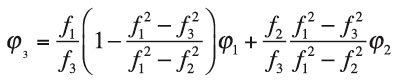where:
φ1 , f1 are the phase and frequency of the first signal in cycles and Hz, respectively
φ2 , f2   are the phase and frequency of the second signal in cycles and Hz, respectively
φ3 , f3   are the phase and frequency of the third signal in cycles and Hz, respectively.

It was confirmed that for all pass periods (elevation angles less than 10 degrees were not tested), the difference between the calculated phase and real phase was always less than one-tenth of a cycle. GPS Block IIF satellites PRN 1 and PRN 25 were used to prove this: the L1 C/A-code and L5 signals were used as the first and second signals, with the L2C signal as the third unknown.

If two known signals are not available, and the ionospheric delay cannot be precisely calculated, it is theoretically possible to obtain an estimate of the delay from one or more neighboring satellites with two signals available. Calculations and estimations should be carried out to investigate the expected precision.

##### The Experiment

The Galileo E6-B/C signal as currently transmitted by the IOV satellites was selected for the experiment, as its structure has not been published. The E6 signal has three components: E6-A, E6-B and E6-C. The E6-A component is part of the Galileo Public Regulated Service, while the two other components will serve the Galileo Commercial Service. The E6-B component carries a data signal, while the E6-C component is a pilot signal.

From open sources, it is known that the carrier frequency of the E6 signal is 1278.75 MHz and that the E6-B and E6-C components use BPSK modulation at 5,115 chips per millisecond with a primary code length of one millisecond. E6-B’s data rate is 1,000 bits per second and the total length of the pilot code is 100 milliseconds (a secondary code of 100 bits over 100 milliseconds is also present in the E6-C signal, which aids in signal acquisition).

A slightly modified commercial high-precision multi-GNSS receiver, with the E6 band and without the GLONASS L2 band, was used for this experiment. The receiver was connected to a conventional GNSS antenna, placed on a roof and was configured as described above. The E1 signal was used as the first signal and E5a as the second signal. The E6 code tracking (using 5,115 chip values of zero) was 100 percent guided from the E1 code tracking (the changing of the code delay in the ionosphere was ignored). The E6 phase was guided from E1 and E5a using the above equation. Two arrays for 511,500 I and Q samples were organized in firmware. The integration period was set to one chip (200 nanoseconds).

Galileo IOV satellite PRN 11 (also variously known as E11, ProtoFlight Model and GSAT0101) was used initially, and the experiment started when the satellite’s elevation angle was about 60 degrees and lasted for only about 30 minutes. Then the I and Q vectors were downloaded to a PC and analyzed.

##### Decoding of Pilot Signal (E6-C)

Decoding of the pilot signal is made under the assumption that any possible influence of the data signal is small because the number of ones and zeros of E6-B in each of 511,500 chips of the 100-millisecond integration interval is about the same. First, the secondary code was obtained. Figure 1 shows the correlation of the first 5,115 chips with 5,115 chips shifted by 0 to 511,500 chips. Because the initial phase of the E6 signal is unknown, two hypotheses for computing the amplitude or signal level were checked: [A] = [I] + [Q] and [A] = [I] – [Q], and the combination with the higher correlation value was selected for all further analysis.

In Figure 1, the secondary code is highly visible: we see a sequence of 100 positive and negative correlation peaks (11100000001111 …; interpreting the negative peaks as zeros).This code is the exact complement (all bits reversed) of the published E5a pilot secondary code for this satellite. More will be said about the derived codes and their complements later. It appears that, for all of the IOV satellites, the E6-C secondary codes are the same as the E5a secondary codes.

After obtaining the secondary code, it is possible to coherently add all 100 milliseconds of the integration interval with the secondary code sign to increase the energy in each chip by 100 times. Proceeding, we now have 5,115 chips of the pilot signal ­— the E6-C primary code.

To understand the correctness of the procedure and to check its results, we need to confirm that there is enough signal energy in each chip. To this end, a histogram of the pilot signal chip amplitudes can be plotted (see Figure 2). We see that there is nothing in the middle of the plot. This means that all 5,115 chips are correct, and there is no chance that even one bit is wrong.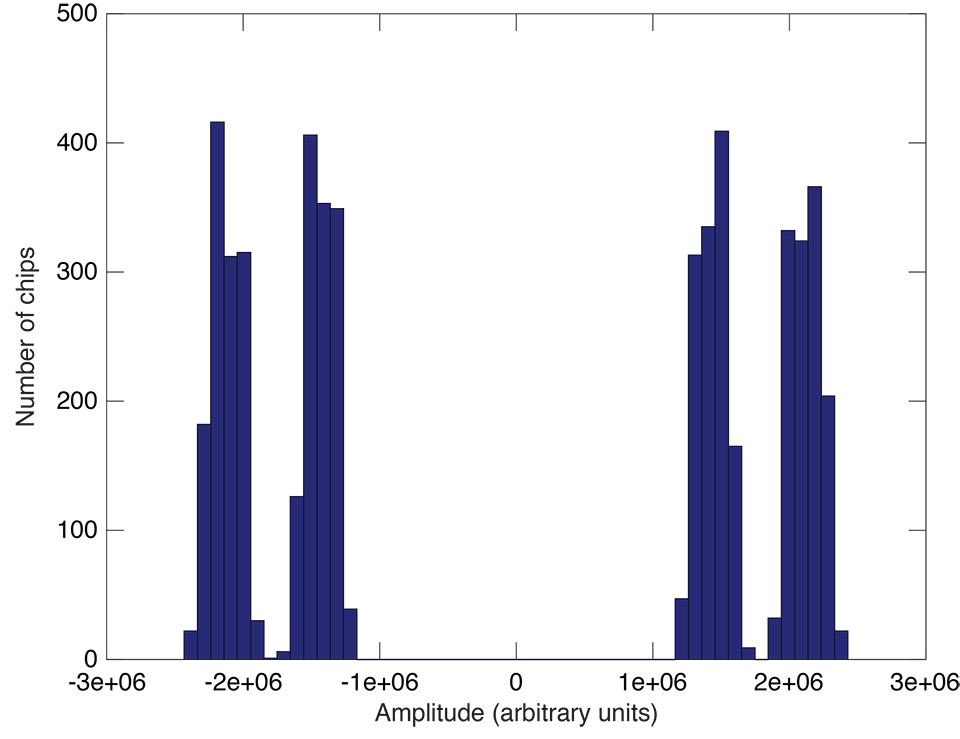Figure 2. Histogram of pilot signal chip amplitude in arbitrary units.

But there is one effect that seems strange at first glance: instead of two peaks we have four (two near each other). We will shortly see that this phenomenon results from the influence of the E6-B data signal and it may be decoded also.

##### Decoding the Data Signal

The presence of four peaks in the histogram of Figure 2 was not understood initially, so a plot of all 511,500 signal code chips was made (see Figure 3).
Interestingly, each millisecond of the signal has its own distribution, and milliseconds can be found where the distribution is close to that when two signals with the same chip rate are present. In this case, there should be three peaks in the energy (signal strength) spectrum: –2E, 0, and +2E, where E is the energy of one signal (assuming the B and C signals have the same strength).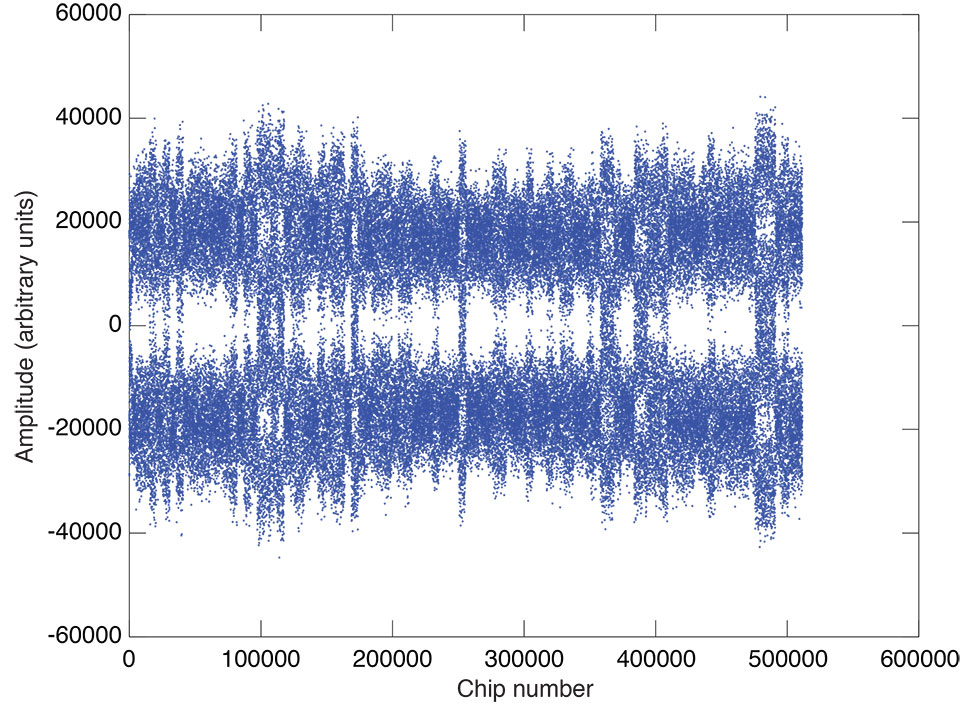Figure 3. Plot of 511,500 signal code chip amplitudes in arbitrary units.

One such time interval (starting at millisecond 92 and ending at millisecond 97) is shown in Figure 4. The middle of the plot (milliseconds 93 to 96) shows the described behavior. Figure 5 is a histogram of signal code chip amplitude for the signal from milliseconds 93 to 96.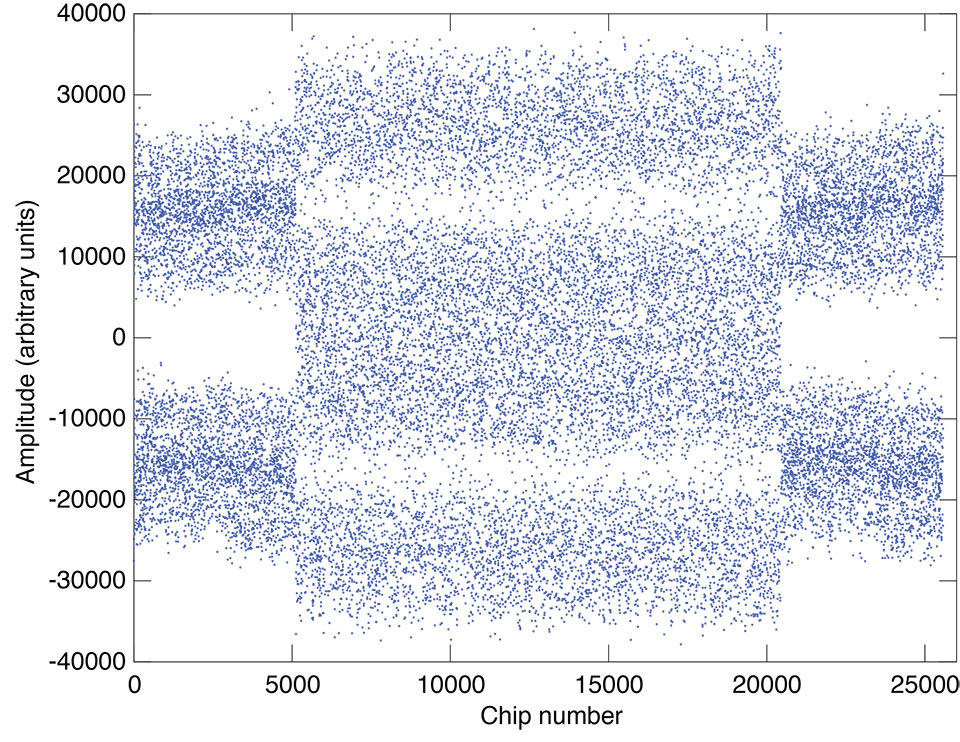Figure 4. Plot of signal code chip amplitude in arbitrary units from milliseconds 93 to 96.

Then we collect all such samples (milliseconds) with the same data sign together to increase the signal level. Finally, 5,115 values are obtained. Their distribution is shown in Figure 6.

The central peak is divided into two peaks (because of the presence of the pilot signal), but a gap between the central and side peaks (unlike the case of Figure 5) is achieved. This allows us to get the correct sign of all data signal chips. Subtracting the already known pilot signal chips, we get the 5,115 chips of the data signal — the E6-B primary code. This method works when there are at least some samples (milliseconds) where the number of chips with the same data bit in the data signal is significantly more than half.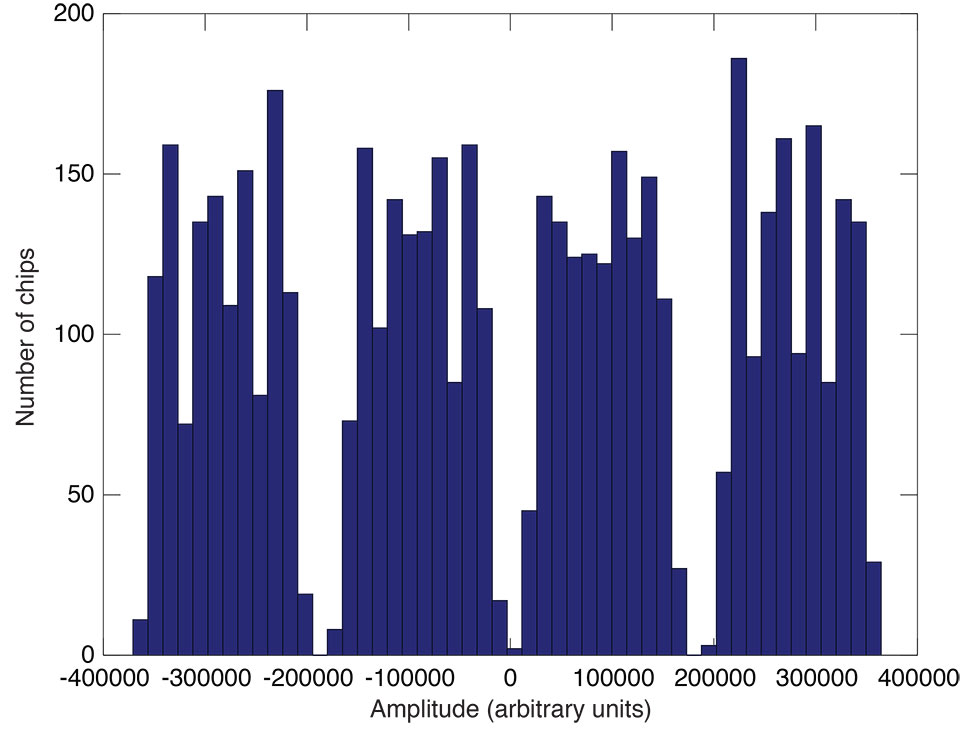Figure 6. Histogram of the signed sum of milliseconds chip amplitude with a noticeable presence of the data signal.

##### Proving the Codes

The experimentally determined E6-B and E6-C primary codes and the E6-C secondary codes for all four IOVsatellites (PRNs 11, 12, 19, and 20) were put in the receiver firmware. The receiver was then able to autonomously track the E6-B and E6-C signals of the satellites.

Initial decoding of E6-B navigation data has been performed. It appears that the data has the same preamble (the 16-bit synchronization word) as that given for the E6-B signal in the GIOVE Interface Control Document (ICD). Convolutional encoding for forward error correction is applied as described in the Galileo Open Service ICD, and 24-bit cyclic redundancy check error detection (CRC-24) is used. At the time of the analysis, all four IOV satellites transmitted the same constant navigation data message.

Plots of PRN 11 E6 signal tracking are shown in Figure 7 and in Figure 8. The determined codes may be found at www.gpsworld.com/galileo-E6-codes. Some of these codes may be the exact complement of the official codes since the code-determination technique has a one-half cycle carrier-phase ambiguity resulting in an initial chip value ambiguity. But from the point of view of receiver tracking, this is immaterial.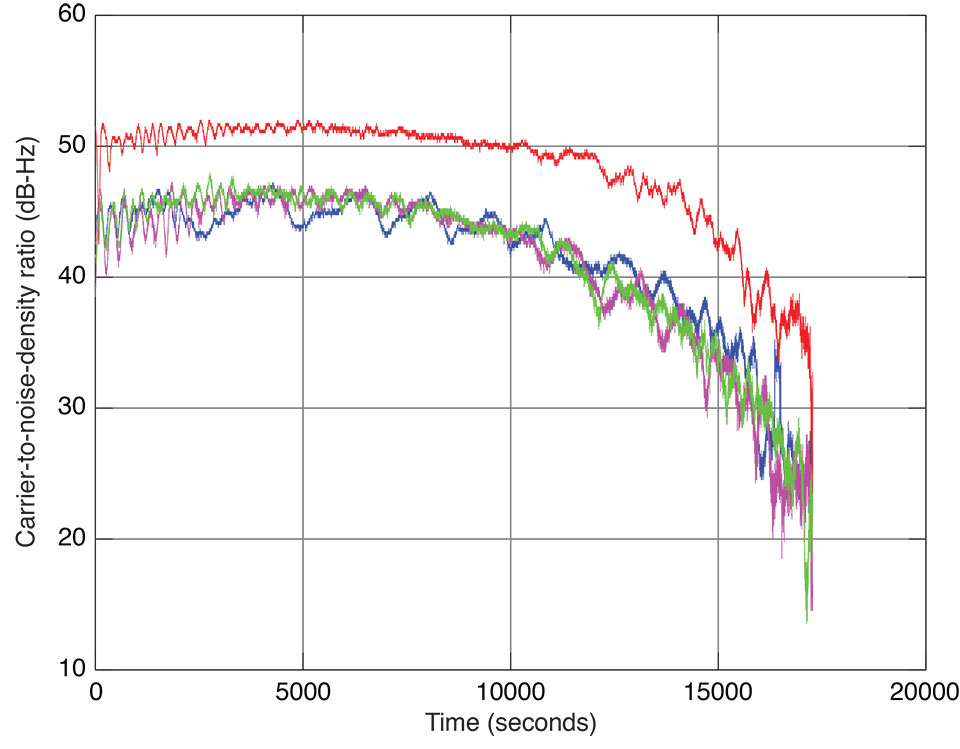Figure 7. Signal-to-noise-density ratio of E1 (red), E5a (magenta), E5b (blue), and E6 (green) code tracking of Galileo IOV satellite PRN 11 on December 21–22, 2012.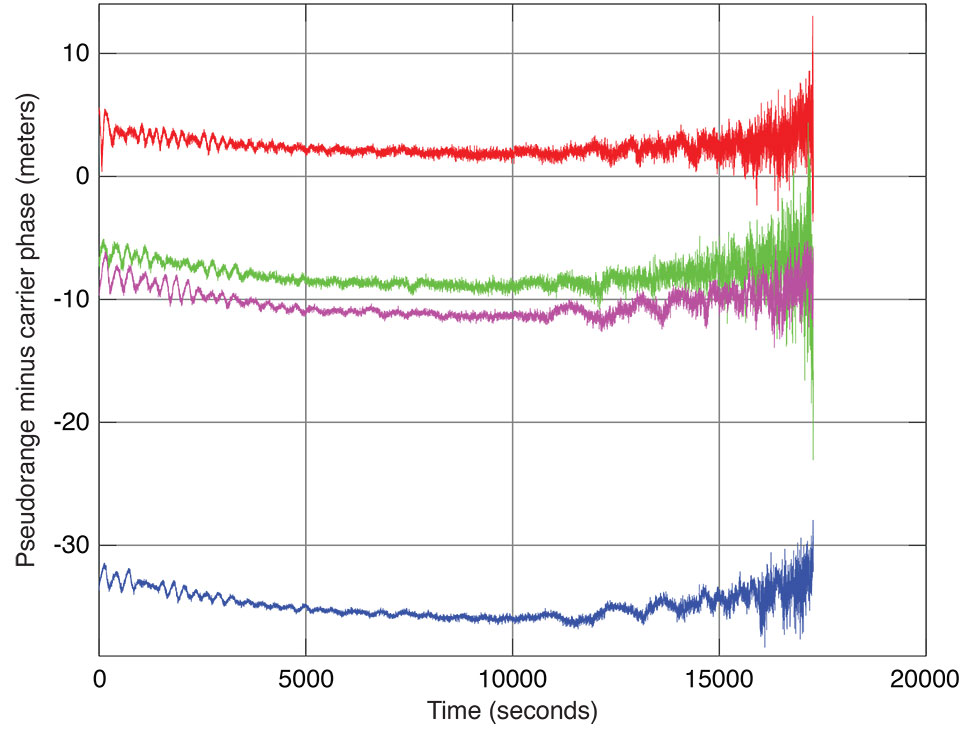Figure 8. Pseudorange minus carrier phase (in units of meters) of E1 (red), E5a (magenta), E5b (blue), and E6 (green) code tracking of Galileo IOV satellite PRN 11 on December 21–22, 2012.

##### Acknowledgments

Special thanks to JAVAD GNSS’s DSP system developers. The system is flexible so it allows us to do tricks like setting the integration period to one chip, and powerful enough to be able to do required jobs within a 200-nanosecond cycle. This article was prepared for publication by Richard Langley.

##### Manufacturers

A JAVAD GNSS TRE-G3T-E OEM receiver, a modification of the TRE-G3T receiver, was used in the experiment, connected to a conventional JAVAD GNSS antenna. Plots of E6 code tracking of all four IOV satellites may be found on the company’s website.

Sergei Yudanov is a senior firmware developer at JAVAD GNSS, Moscow.